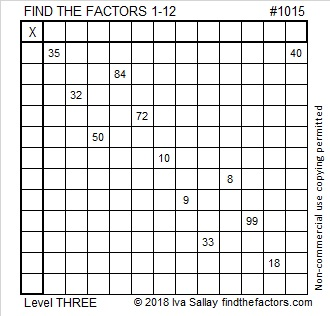# 1015 and Level 3

If you glance at this puzzle for a few seconds, you may think there are three places in the top row where the number 1 will work, but in actuality, only one of those places will work with all the other clues in the puzzle. This is a level 3 puzzle, so start with the top cell in the first column and work down cell by cell placing factors in both the first column and the top row until the puzzle resembles a multiplication table.Print the puzzles or type the solution in this excel file: 12 factors 1012-1018

Here are a few facts about the number 1015:

1² + 2² + 3² + 4² + 5² + 6² + 7² + 8² + 9² + 10² + 11² + 12² + 13² + 14² = 1015. That makes 1015 the 14th square pyramidal number.

331 + 337 + 347 = 1015. That’s the sum of 3 consecutive prime numbers.

1015 is the hypotenuse of four Pythagorean triples:
119-1008-1015
168-1001-1015
609-812-1015
700-735-1015

1015 looks interesting when it is written is some other bases:
707 in BASE 12
1D1 in BASE 26 (D is 13 base 10)
TT in BASE 34 (T is 29 base 10)
T0 in BASE 35

• 1015 is a composite number.
• Prime factorization: 1015 = 5 × 7 × 29
• The exponents in the prime factorization are 1, 1, and 1. Adding one to each and multiplying we get (1 + 1)(1 + 1)(1 + 1) = 2 × 2 × 2 = 8. Therefore 1015 has exactly 8 factors.
• Factors of 1015: 1, 5, 7, 29, 35, 145, 203, 1015
• Factor pairs: 1015 = 1 × 1015, 5 × 203, 7 × 145, or 29 × 35
• 1015 has no square factors that allow its square root to be simplified. √1015 ≈ 31.85906